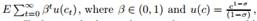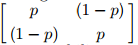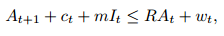# Mobility Costs Bertola A Worker Seeks To Maximize And E Is The Expectation Operator 1946872

Mobility costs (Bertola) A worker seeks to maximizeand E is the expectation operator. Each period, the worker supplies one unit of labor inelastically (there is no unemployment) and either wg or wb, where wg > wb. A new “job” starts off paying wg the first period. Thereafter, a job earns a wage governed by the two-state Markov process governing transition between good and bad wages on all jobs; the transition matrix isA new (well-paying) job is always available, but the worker must pay mobility cost m > 0 to change jobs. The mobility cost is paid at the beginning of the period that a worker decides to move. The worker’s period-t budget constraint iswhere R is a gross interest rate on assets, ct is consumption at t, m > 0 is moving costs, It is an indicator equaling 1 if the worker moves in period t, zero otherwise, and wt is the wage. Assume that A0 > 0 is given and that the worker faces the no-borrowing constraint, At ≥ 0 for all t.

a. Formulate the Bellman equation for the worker.

b. Write a Matlab program to solve the worker’s Bellman equation. Show the optimal decision rules computed for the following parameter values: m = .9, p = .8, R = 1.02, β = .95, wg = 1.4, wb = 1, σ = 4. Use a range of assets levels of [0, 3]. Describe how the decision to move depends on wealth.

c. Compute the Markov chain governing the transition of the individual’s state (A, w). If it exists, compute the invariant distribution.

d. In the fashion of Bewley, use the invariant distribution computed in part c to describe the distribution of wealth across a large number of workers all facing this same optimum problem.4.6/5

Price (USD)
\$